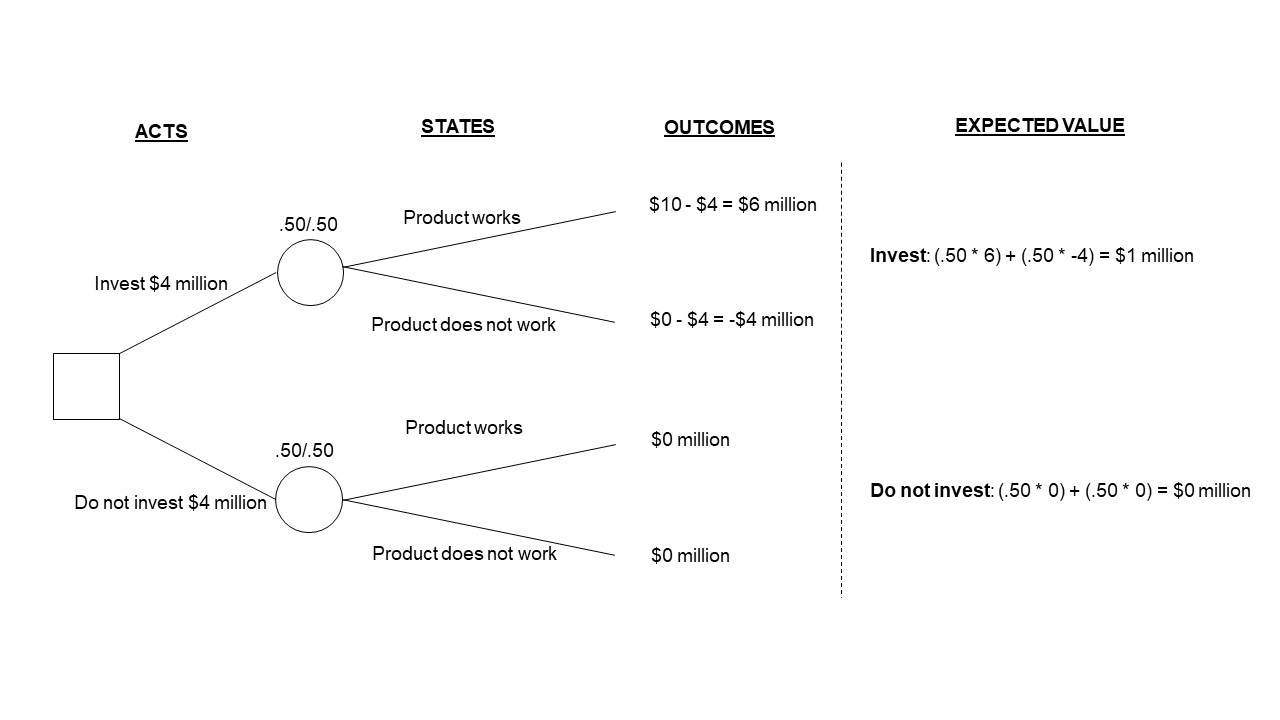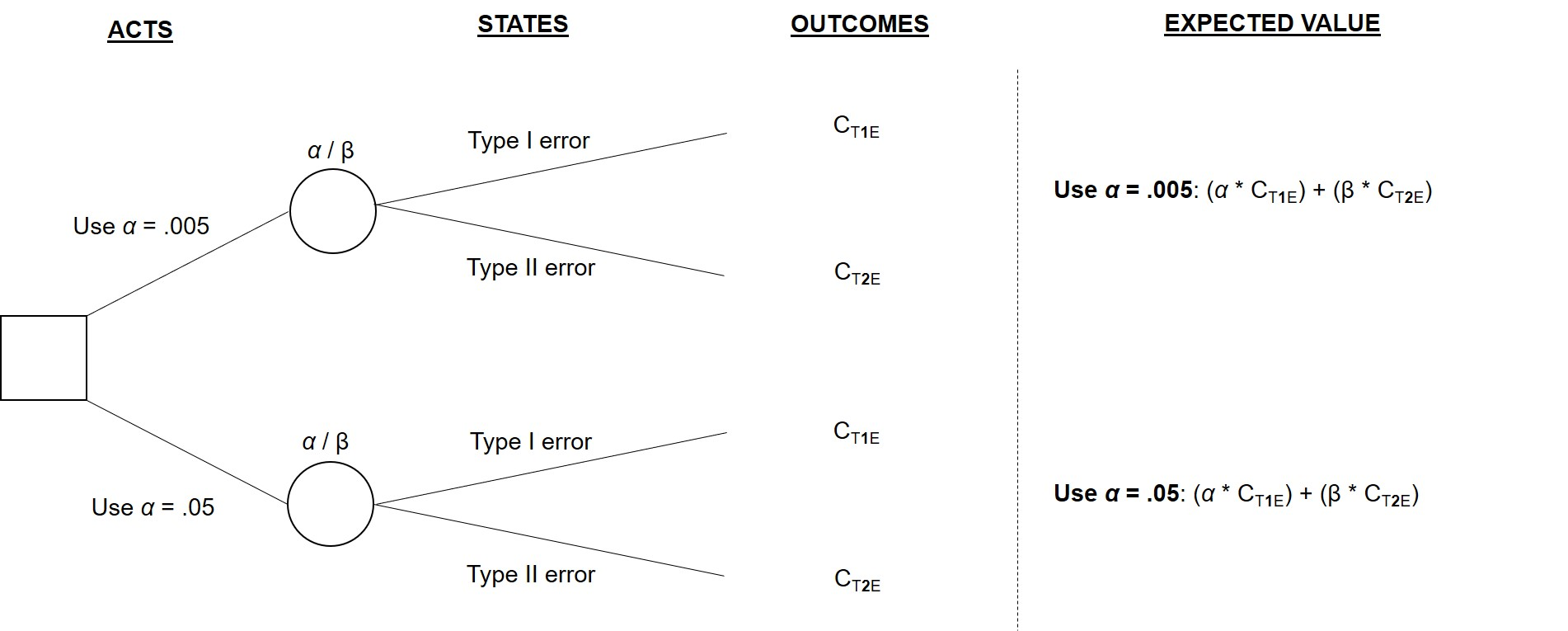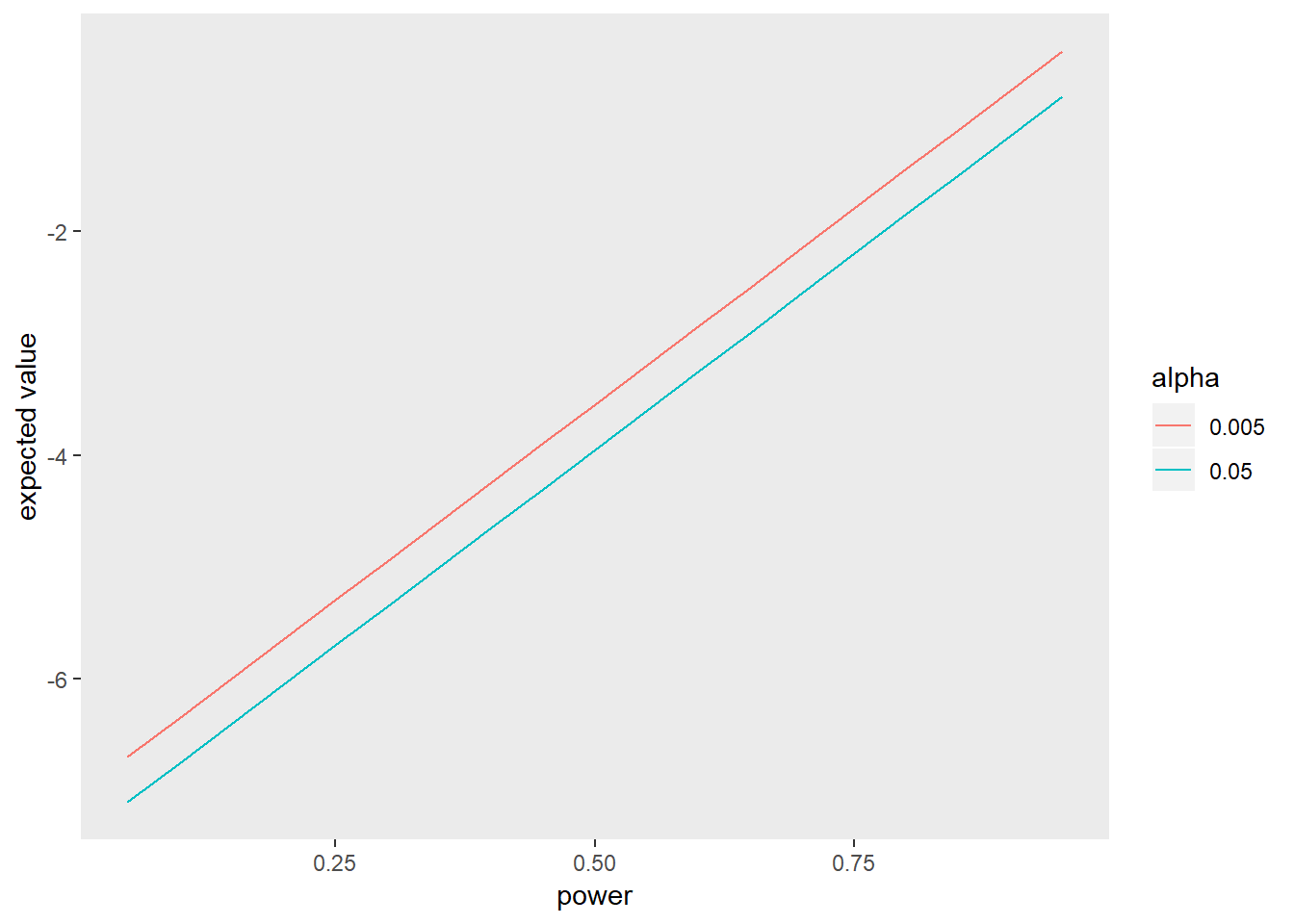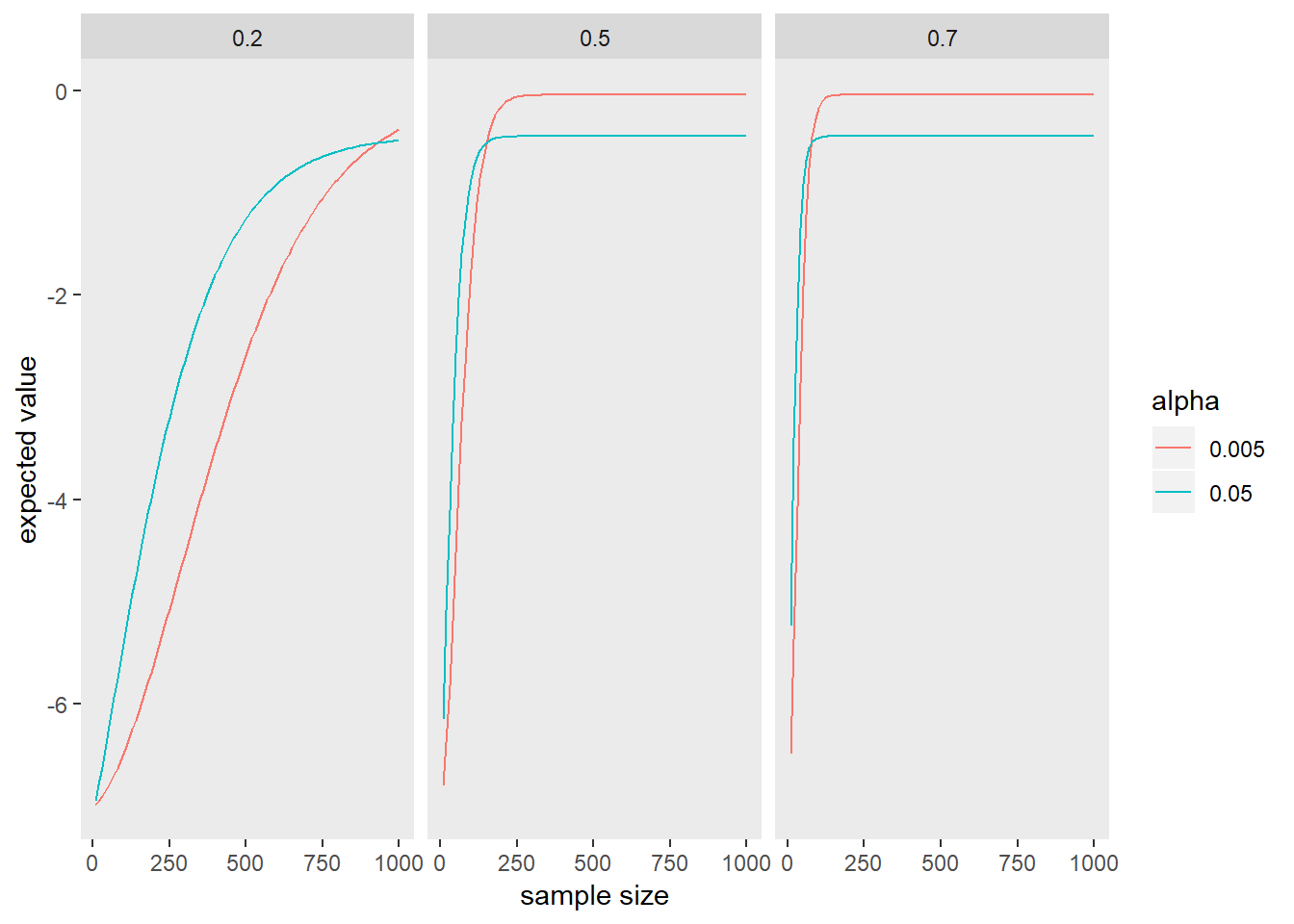# The 20% Statistician

A blog on statistics, methods, philosophy of science, and open science. Understanding 20% of statistics will improve 80% of your inferences.

## Monday, July 15, 2019

### Using Decision Theory to Justify Your Alpha

Recently, social scientists have begun to critically re-examine their most sacred (yet knowingly arbitrary) traditions: = .05. This reflection was prompted by 72 researchers (Benjamin et al., 2017) who argued that researchers who use Null Hypothesis Significance Testing should redefine significance criteria to = .005 when claiming the discovery of a new effect. Their rationale is that p-values near .05 often provide only weak evidence for the alternative hypothesis from a Bayesian perspective. Furthermore, from a Bayesian perspective, if one assumes that most alternative hypotheses are wrong (an assumption they justify based on prediction markets and replication results), p-values near .05 often provide evidence in favor of the null hypothesis. Consequently, Benjamin and colleagues suggest that redefining statistical significance to = .005 can limit the frequency of non-replicable effects in the social science literature (i.e., Type 1 errors).

In a reply to Benjamin and colleagues, Lakens and colleagues (2018) argued that researchers should not constrain themselves to a single significance criterion. Instead, they suggested that researchers should use different significance criteria, so long as they justify their decision prior to collecting data. Intuitively, we can think of real-world scenarios where this makes sense. For example, when screening for cancer, we allow more false positives in order to ensure that real cancer cases are rarely missed (i.e., larger s). On the other hand, many courts of law try to strictly limit how often individuals are wrongly convicted (i.e., smaller s). Nevertheless, if we accept Lakens and colleagues’ proposal, we are left with a more difficult questions: How can we justify our alphas? I suggest that the answer lies in decision theory.

## A simple overview of decision theory: Making rational decisions under risk

Before using decision theory to justify alphas, it is helpful to first review decision theory in a more classic domain: financial decision making. Figure 1 is an illustration of a hypothetical investment decision where you must decide whether to invest $4 million in the development of a product. The so-called decision tree in Figure 1 has three major components: 1. Acts: Acts are the possible behaviors related to the decision. In this example, you either invest$4 million or do not invest in the product.
2. States: States represent the possible truths of the world as it relates to the decision-making context. To simplify this example, we will assume that there are only two relevant states: the product works or the product does not work. In this example, lets assume that we know there is a 50% chance that the product will work.
3. Outcomes: Outcomes are the consequences of each potential state. In this example, if you decide to invest and the product works, you receive a 6 million return on your investment. If you invest and the product does not work, you lose 4 million. If you abstain from investing, you neither gain nor lose money regardless of whether the product works.

To be a rational decision maker, you should choose whichever act maximizes your expected value. The expected value of each act is calculated by taking the sum of the probability-weighted value of each potential outcome. Whichever act has higher expected value is considered the rational choice, and the law of large numbers dictates that you will be better off in the long run if you act in a manner that maximizes your expected value. In this example, although the investment is risky, you should typically invest because the expected value of investing exceeds the expected value of not investing.Figure 1. Decision tree for a simple investment decision

## Evaluating significance criteria using decision theory

Figure 2 illustrates a decision tree that formalizes the decision to use α = .05 or α = .005. In order to evaluate which significance criterion to adopt, we need to consider not only the Type 1 error rate (i.e., α) but also the Type 2 error rate (i.e., 1 - β). This is because, all else equal, lowering the Type 1 error rate increases the Type 2 error rate.Figure 2. Simplified decision tree for comparing statistical significance criteria

To calculate the expected value of adopting each significance criterion, researchers need to specify the costs of Type 1 and Type 2 errors. These costs are denoted in Figure 2 as (cost of Type 1 error) and (cost of Type 2 error). Like the investment example, we could operationalize cost in terms of money. However, in the following examples, I will operationalize cost on a unit-less continuous 10-point scale. (This is an inconsequential matter of preference.) In this post, I will specify the cost of a Type I error as -9 out of 10 (i.e., = -9) and the cost of a Type II error as -7 out of 10 (i.e., = -7). However, costs could, of course, vary based on the research context.

Just like the investment example, the expected value of using each significance criterion is calculated using the sum of the probability-weighted cost of each potential outcome.

ExpVal <- function
(alpha, pwr, CT1E = -9, CT2E = -7){
(alpha * CT1E) + ((1 - pwr) * CT2E)
}

### Example 1: Comparing significance criteria with power held constant

First, we will examine the expected value of using α = .05 vs. α= .005 when power is held constant at .80.

# Expected value of using alpha = .05
ExpVal(alpha = .05, pwr = .80)
##  -1.85
# Expected value of using alpha = .005
ExpVal(alpha = .005, pwr = .80)
##  -1.445
When power is held constant at .80, results indicate that it is rational to adopt α = .005 vs. α .05. This is perhaps not surprising; If power is held constant, it is always more rational to adopt stricter α values (assuming, of course, that you place negative value on committing errors). This is illustrated below.This figure demonstrates that, when power is constant, = .005 is more rational than = .05. However, given that stricter significance thresholds are always more rational when power is constant, = .005 is less rational than = .001, and = .001 is less rational than = 5 x . Unfortunately, social scientists cannot adopt an infinitely small significance threshold because we do not have infinite participants and resources. Since power is directly related to sample size, researchers with a set number of participants achieve less power when they adopt stricter significance thresholds. Consequently, determining the expected value of adopting stricter significance thresholds requires researchers to determine how much power they can achieve (i.e., their sample size, effect size, and research design) and how much value they place on Type 1 and Type 2 errors.

### Example 2: Comparing alphas based on sample size, effect size, and costs assigned to errors

The figure below compares the expected value of using α = .005 vs. α = .05 in a two-group between-subject design when (a) CT1E = -9, (b) CT1E = -7, and (c) the alternative hypothesis is two-sided. This figure illustrates that the expected value of a significance threshold depends on power—i.e., both sample size and the size of the underlying effect. For example, when examining small effects, it is rational to use α = .05 instead of α = .005 until the sample exceeds 930. However, if one is examining a medium-sized effect, it becomes rationale to use α = .005 once the sample exceeds just 160. We could also use this figure to compare the expected value when different sample sizes are used for each criterion. For example, if one has the option to collect 100 participants with α = .05 or collect 220 participant with α = .005, it is rational under this formalization to adopt the stricter α= .005 threshold.This figure contains a lot of information, but the main takeaway is that the expected value of adopting stricter significance thresholds requires researchers to determine how much power they can achieve (which depends on sample size, effect size, and experimental design. Put simply, Figure 4 demonstrates that sometimes it is more rational to use = .05 instead of = .005, and other times it is not.

### Example 3: Choosing optimal alpha values

So far, we have used a decision theory framework to compare just two alpha values. However, in theory, we should consider all potential alpha values in order to determine which one maximizes our expected value. This is just what the optimize function in R allows us to do. Imagine you are examining a small effect using a two-group between-subjects design, but only have time to collect 100 participants. What alpha value should you use to maximize your expected value? Using the optimize function, the code below demonstrates that the answer is approximately = 0.18. On the other hand, if you can collect 200 participants, the most rational alpha to adopt is .14. You can adjust the power function to further explore how the experimental design, sample size, effect size, and alternative hypothesis influence the optimal alpha value.

optimize(f = function(alpha){
ExpVal(alpha = alpha,
pwr = pwr.t.test(n = 100, d = .20,
sig.level = alpha, power = NULL,
type = "two.sample",
alternative = "two.sided")\$power)
}
, interval = c(0,1), maximum = TRUE)

### Limitations of the framework presented here

The framework I present here provides a simple illustration of how decision theory can be used to justify alphas. However, in the interest of keeping the framework simple, I introduced at least four limitations.
First, the framework does not currently highlight how researchers could formally specify the costs of Type 1 and 2 errors. Choosing a number of a Likert-type scale is a simple approach. However, decision theorists often specify more complex loss functions, wherein they identify the various factors that influence the cost of a state. Second, this framework currently assumes that researchers are uninterested in the prior probability that the alternative and null hypotheses are true. In order to incorporate these priors, Bayesian Decision Theory is an excellent alternative. Third, this framework helps specify what is rational for the individual, but not necessarily what is rational for the scientific community. For example, an individual researcher may not care so much about committing a Type 1 error (i.e., they might assign a low negative value). However, Type I errors may be more costly for the scientific community, as significant resources may be spent chasing and correcting the Type I error. When considering what is rational for the scientific community, researchers will have to consider more complex decision theory frameworks, such as game theory. Fourth, this framework does not current specify what is rational in scenarios where researchers plan to conduct multiple studies. For example, researchers may assign lower cost to committing Type 2 errors if they plan to conduct pooled or meta-analyses after several studies. Nevertheless, decision theory frameworks can be easily expanded to evaluate multi-step decision making problems.

## Conclusion

In a decision theory framework, justifying your alpha is an act where you strive to maximize expected value. This differs from other proposed approaches to justifying alphas. For example, in a previous blog post, Lakens discussed that researchers could justify alphas in a way that (1) minimizes the total combined error rate (i.e., Type 1 + Type 2 error), or (2) balances error rates. Although outside of the scope of this blog post, there are scenarios where both of Lakens’ proposed approaches are not rational (i.e., do not maximize expected value). When we use decision theory, on the other hand, we can ensure that our decisions always maximize our expected value.
I agree with Benjamin et al. (2017) that p-values near .05 can provide weak evidence for an alternative hypothesis. I also agree that changing to .005 could potentially reduce the number of Type 1 errors in the literature. However, I do not believe that strictly adopting = .005 (or even = .05) is rational. Rather, I agree with Lakens and colleagues’ (2017) call to “justify your alpha”, and I argue that decision theory provides an ideal framework for formalizing these justifications. In the simple decision theory framework I presented here, the expected value of using a significance criterion depends on (1) the probability of committing a Type 1 error, (2) the perceived cost of a Type 1 error, (3) the probability of committing a Type 2 error (i.e., power, which requires knowledge of sample size, effect size, and research design), and (4) the perceived cost of Type 2 errors.

Consequently, depending on obtainable power and the costs assigned to errors, the most rational significance criterion will vary in different experimental contexts.

Some researchers may feel uncomfortable with such a flexible approach to defining statistical significance and argue that the field needs a clear significance criterion to maintain order. Although I feel that flexible statistical criterion is the most valid way to engage in null hypothesis significance testing, I concede there may be practical benefits to establishing a single significance criterion, or even a few different significance criteria. (Ultimately, though, this is a question that can be answered by—you guessed it—decision theory!) Through critical discussion, perhaps scientists will agree that they are willing to sacrifice nuanced rationality in the name of simpler guidelines for significance testing. If this is the case, we should still use decision theory to formally justify what this criterion should be. In order to do so, researchers will need to specify (a) the average effect size of interest, (b) the average achievable sample size, (c) the typical experimental design, and (d) the average costs of Type 1 and 2 errors.

Whether or not researchers decide to use flexible significance criterion, multiple significance criterion, or a single significance criterion, we should not arbitrarily define statistical significance. Instead, we should rationalize statistical significance using a decision theory framework.

#### 3 comments:

1.Thanks Daniel, it's good to hear an informed opinion which I see as a gentle push away from using the same significance threshold for all kinds of tests in a discipline, or even in sciences as a whole. This has always perplexed me as I'm mostly working in business settings where risks and rewards can be estimated with a fair degree of precision since the number of people/situations affected by a given inference is more or less limited, unlike the sciences.

I've actually worked on arriving at significance thresholds and sample sizes (and therefore power/minimum effect of interest) which achieve optimal balance of risk and reward for an online controlled experiment based on its particular circumstances. A brief description of my work can be found at http://blog.analytics-toolkit.com/2017/risk-vs-reward-ab-tests-ab-testing-risk-management/ while a more detailed expose will soon be released in my upcoming book where I devote a solid 30 pages to the topic ( https://www.abtestingstats.com/ ), for anyone interested.

2.Either I've misunderstood this, or there's something wrong with it or missing from it. The decision tree in Figure 1 is fine, but the tree in Figure 2 isn't analogous to it. In Fig 1, you make the decision whether or not to invest, and then the chance nodes show all the possible outcomes - the product works, or it doesn't - and the probabilities of those are their unconditional probabilities, 0.5 and 0.5 for each. In Figure 2, you choose the alpha, but the following chance nodes don't include all the possible outcomes. They only include the possibilities that there is a type 1 or a type 2 error, but there's another possibility, that there's no error at all and the test gives the correct outcome. Also, the probabilities assigned to the two error types are conditional - alpha is the probability of a result in the critical region (i.e. 'significant') conditional on the null hypothesis being correct, that is, conditional on the true effect being zero, and beta is the probability of a result outside the critical region (i.e. 'not significant'), conditional on the true effect being non-zero, so you can't just put them both in the same expected value calculation like that, as you then find the expected value from two different probability distributions that are conditional on different things, which makes no sense (to me at least).

In the Figure 1 example there are only two states (product works or not), but in the testing example there are four:
(i) There is no true effect (null hypothesis true) and test result non-significant.
(ii) There is no true effect and test result is significant
(iii) There is a true effect (null hypothesis false) and test result non-significant.
(iv) There is a true effect and test result is significant.

Or you could draw a tree with two sets of chance nodes, one set for whether the null hypothesis is true, and one, which could then be conditional on the first node, for whether the test result is significant or not. Then the probabilities for the second set would be alpha, 1 - alpha for those following "Null hypothesis true", and 1 - beta, beta, for those following "Null hypothesis not true". That would work, but you still have to specify the probabilities on the first set of nodes, that is, the probability of whether the null hypothesis is true, and that is the prior probability that you want to avoid. But I don't think you can avoid it - if you put in all four outcomes on the chance nodes and work out their probabilities, that involves the probability that the null is true, that is, the prior.

You might be able to take a different decision theoretic approach that avoids using the prior probabilities, but the one you've used, with decision trees, is pretty weell inevitably Bayesian, I think.

3.Hi

Not a statistician, instead a physician trying to learn statistics.
A bit tired today, so perhaps misunderstood something.

I think I have some comments to this. Interesting about decision theory, though.

Here goes:
In finance it’s clear whether your result is “good” or “bad”/”true” or “false”. You have an economic return or loss on a certain level.
In for example science (but also in medicine) you get a result either way.
The “value” lies (somewhat) in whether you can trust the result or not.
The “return” or “loss” could perhaps be seen as whether the applications of the results turn out to be useful in practice or not.

I’m not sure that evaluation through such implementation in general is the best way to go, though.

Instead I think one could start with setting a level of certainty that’s needed when comes to deeming a scientific question answered or not answered.
When claiming a scientific hypothesis is answered - what is the acceptable likelihood that the answer we have is a false nullresult, or a false positive result? (Either for a single hypothesis, or in general, for a number of them.)
Here I think decision theory may have it’s place: What the “sought for” level of certainty should be in a given situation (with given economic restraints, etc), or in the scientific community as a whole, can probably be examined with some form of decision theory - that in combination with known facts, etc.

The levels of certainty perhaps don’t have to be stated in numbers.
Perhaps “very highly likely” or “very, very unlikely” are good enough.

Then, when one knows that level of requested certainty, one can probably use a stepwise process, to reach it.
This similar to a “stepwise diagnostic process” in medicine or psychology that I think you are familiar with, where you often use several test in a row. - In science being equivalent to several studies in a row for a given hypothesis.
There, in general, depending on level of prior probability, etc, I think it may be smart to go for an appropriate level of beta or alpha, to obtain the requested level of certainty for either nulls or positives, in a first run, and then examine either positives or nulls further, depending on which category that is known to contain to many false ones.
- Perhaps similar to Bayesian decision theory that you mention.

This could probably be tested with some sort of simulation.

I may be wrong, but I think that is a somewhat easier approach than the one you propose.

(Perhaps also a bit more informative or effective.
I think it’s better in the long run to know that 3 % of nullresults, and 25 % of positive ones are probably false, than to know that ca 10 % of each are false. In the first you mostly have to test the positive ones further. In the second you more or less have to test both positives and negatives further.)

Best wishes!